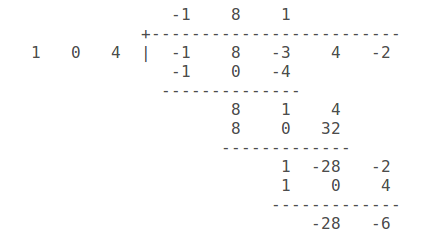# How do you divide (-x^4+8x^3-3x^2+4x-2)/(x^2+4) ?

How do you divide (-x^4+8x^3-3x^2+4x-2)/(x^2+4) ?

Answer 1

You kind of can't.

#### Explanation:

As x^2+4 is not a factor of the numerator, it will never divide out "cleanly".

If all you want to do is simplify the equation, you can rewrite the denominator as a (x^2+4)^-1, and use the distributive property to multiply it through.

If you plug the equation into wolfram alpha, it will show you how futile the attempt to simplify the equation will be.

http://www.wolframalpha.com/input/?i=factor+%28%E2%88%92x%5E4+%2B+8x%5E3+%E2%88%92+3x%5E2+%2B+4x+%E2%88%92+2%29%2F%28x%5E2%2B4%29

If you know anything about Galois theory, then you can factor this over finite fields with a prime number of elements; however, that answer I suspect exceeds the scope of the question.

Long divide the coefficients to find:

$\frac{- {x}^{4} + 8 {x}^{3} - 3 {x}^{2} + 4 x - 2}{{x}^{2} + 4} = - {x}^{2} + 8 x + 1 + \frac{- 28 x - 6}{{x}^{2} + 4}$

#### Explanation:

I like to long divide the coefficients, not forgetting to include $0$ for any missing power of $x$...The process is similar to long division of numbers.

For a more fully explained example, see: http://socratic.org/questions/how-do-you-divide-4x-4-12x-3-5x-3-x-2-4x-4

We find:

$\frac{- {x}^{4} + 8 {x}^{3} - 3 {x}^{2} + 4 x - 2}{{x}^{2} + 4}$

$= - {x}^{2} + 8 x + 1 + \frac{- 28 x - 6}{{x}^{2} + 4}$

Or if you prefer:

$- {x}^{4} + 8 {x}^{3} - 3 {x}^{2} + 4 x - 2$

$= \left({x}^{2} + 4\right) \left(- {x}^{2} + 8 x + 1\right) + \left(- 28 x - 6\right)$

#### Earn Coin

Coins can be redeemed for fabulous gifts.

Similar Homework Help Questions

• ### How do you divide (-x^4-4x^3-3x^2-6x-2)/(x^2+4) ?